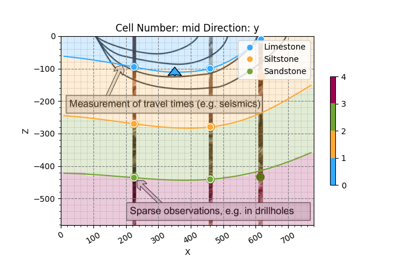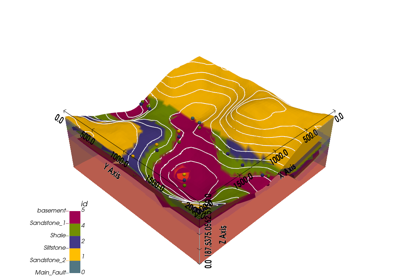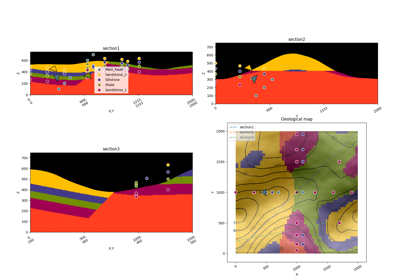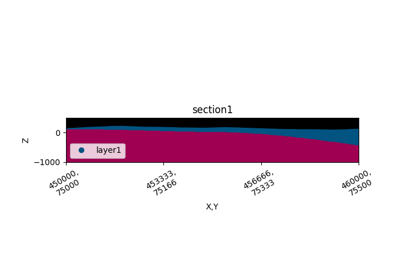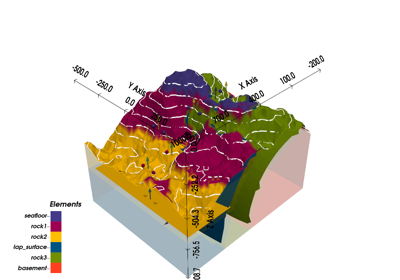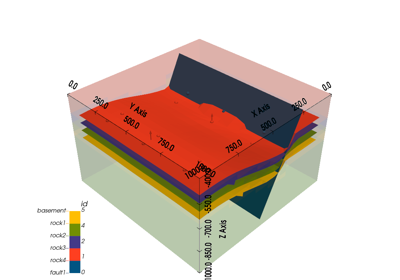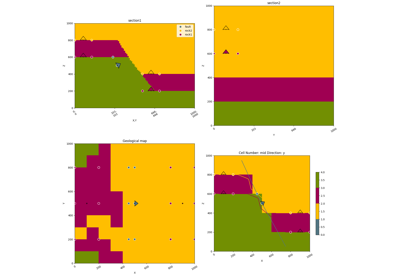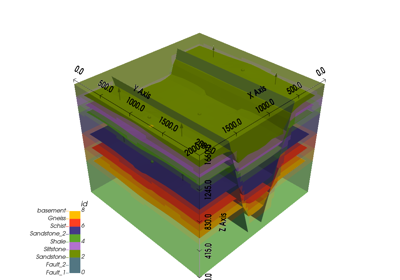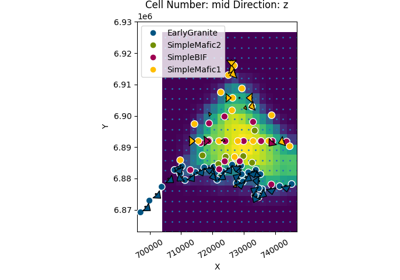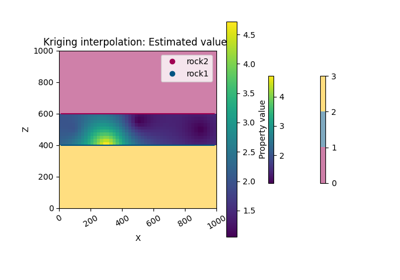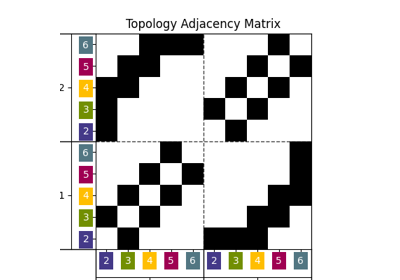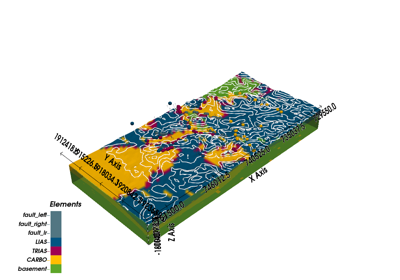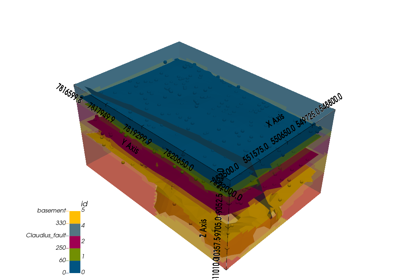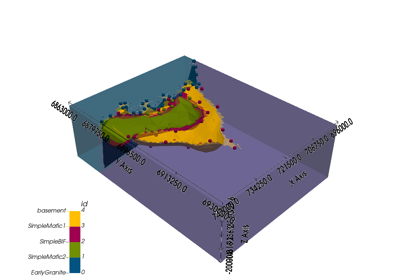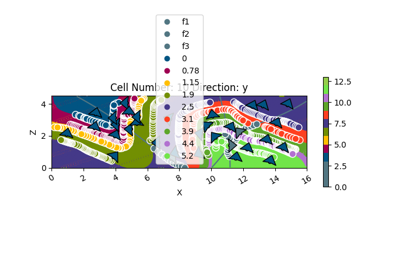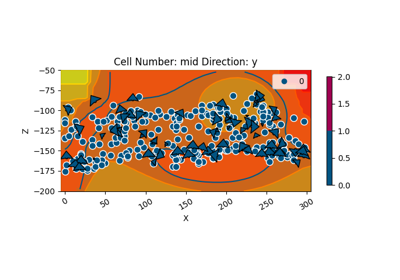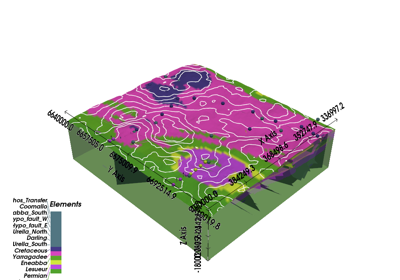# gempy.compute_model¶

gempy.compute_model(model: gempy.core.model.Project, output=None, at: Optional[numpy.ndarray] = None, compute_mesh=True, reset_weights=False, reset_scalar=False, reset_block=False, sort_surfaces=True, debug=False, set_solutions=True, **kwargs)gempy.core.solution.Solution[source]

Computes the geological model and any extra output given in the additional data option.

Parameters
• model (Project) – [s0] Container class of all objects that constitute a GemPy model.

• output (str {'geology', 'gravity'}) – Compute the lithologies or gravity

• at (np.ndarray) –

• compute_mesh (bool) – if True compute marching cubes: [s1] Compute the surface (vertices and edges) of a given surface by computing marching cubes (by skimage)

• reset_weights (bool) – Not Implemented

• reset_scalar (bool) – Not Implemented

• reset_block (bool) – Not Implemented

• sort_surfaces (bool) – if True call Project.set_surface_order_from_solution: [s2] Order the surfaces respect the last computation. Therefore if you call this method, after sorting surface_points without recomputing you may get wrong results.

• debug (bool) – if True, the computed interpolation are not stored in any object but instead returned

• set_solutions (bool) – Default True. If True set the results into the Solutions linked object.

• **kwargs

Keyword Arguments

compute_mesh_options (dict) – options for the marching cube function. 1) rescale: True

Returns

Solutions

## Examples using gempy.compute_model¶